American Journal of Civil Engineering
Volume 3, Issue 2-2, March 2015, Pages: 80-85

Evaluation of Ten Thousand Cubic Meters Mazut Tank Behavior Against Explosive Charges

Saeid Azmoodeh*, Nasser Arafati

Department of Civil Engineering, University of Tafresh, Tafresh, Iran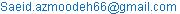(S. Azmoodeh)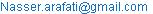(N. Arafati)

Saeid Azmoodeh, Nasser Arafati. Evaluation of Ten Thousand Cubic Meters Mazut Tank Behavior Against Explosive Charges. American Journal of Civil Engineering. Special Issue: Research and Practices of Civil Engineering in Developing Countries. Vol. 3, No. 2-2, 2015, pp. 80-85. doi: 10.11648/j.ajce.s.2015030202.26

Abstract: Considering the terrorist threats and blasts, which may occur to destroy a structure, the effect of explosive on a 10 thousand cubic meter Mazut tank of Mashhad Cement Company is studied. This article studies the behavior of 10 thousand cubic meter tank of storing Mazut with the help of ABQUS3D software. The explosive considered is TNT, which is studied in distances of 15, 25, 50, 100, 200 meters to the structure and with various thicknesses of the tank wall. Finally, the results indicate that by the increase of the wall thickness, the blast effect will mitigate. Also due to the surface wave generated, by the reduction of the blast distance to the structure, the effectiveness of the blast wave will be greater and ultimately, in a distance less than 15 meters, the tank will collapse.

1. Introduction

Considering the terrorist threats and blasts, which may occur to destroy a structure, the effect of explosive on a 10 thousand cubic meter Mazut tank of Mashhad Cement Company is studied.

According to the definition of chapter 21of Iran National structure regulations (Passive defense), explosion is a reaction, in which the burning rate of materials is done with a speed much more than the speed of sound, resulting in the generation of a very high pressure and temperature gradient and the shock wave is generated immediately, which will spread with a high speed . In terms of the classification, the explosion type can be natural, physical, nuclear and chemical. In terms of physical, the explosions caused by the sudden failure of the tanks under pressure or volcanic eruption can be referred. In nuclear explosions it is based on the core separation and the Neutrons and Protons distribution loading, whereas in chemical explosion, the rapid oxidization of elements such as Carbon and Hydrogen can be noted. The explosives can be in the form of solid, liquid and gas, but the explosion of solids has usually the greatest effect in terms of the force released . The explosives can also be classified in terms of the primary and secondary forces, which are exerted on the structures or they can be classified due to the activation of these materials through using condiments such as spark, flame or strike. The secondary stage of an explosion is in fact, the wave generated from that explosion, which can cause a lot of damages to the environment. Among very common explosives, TNT (Tri Nitro Toluene) can be referred.

The explosive, TNT can generate a pressure equal to 300 Kb and a temperature of about 3000 to 4000 Celsius degree. After the explosion the expanded hot gas and a layer of compressed air generate (explosion wave). This wave raises the amount of environment atmospheric pressure at about the gas pressure and the pressure behind the wave will be lower and a relative vacuum due to air intake behind it is generated. This cycle is completed in the next round and it generates the explosive shocks. The pressure exerted by the explosion shocks mitigate with the distance increasing from the explosion source. The pressure graph in terms of distance from the blast site is shown in figure 1 .

Between the years 1928 to 1945 a series of tests were carried out by the headquarters of the Japanese army on the striking blast to a three-storey building with a change in the thickness of the slabs. The result showed that by the increase of the slab thickness in the ceiling of the roof, the intrusion of the bomb inside the building is prevented .

Bing Li & Toe Chien (2008), analyzed two reinforced concrete frames with Finite Elements Method (FEM), which one lacked a wall and the other one was with a wall and compared their dynamic response due to the effect of the distant blast loading with each other. Ehsan Mohtashami & Ahmad Shooshtari (2010)  studied the steel structure behavior as a result of the blast occurring nearby. The existing results indicate that with the increase of the column angles, the improved safety measures and the structure displacement are also reduced. By studying the behavior of concrete constructions against the blast loads, Mohammad Bozorgvar & Ahmad Shooshtari (2011) also concluded that if the building is designed and constructed on the basis of stand-off seismic standards, it must be precisely revised for the stand-off against the blast loads.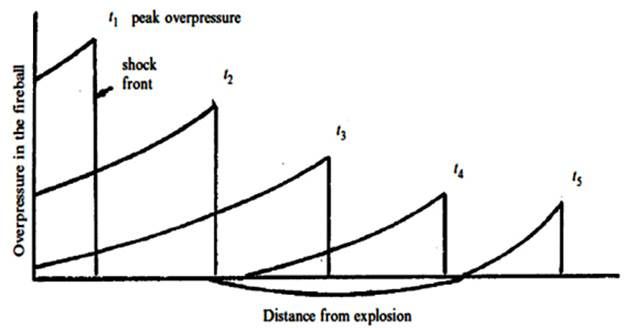Figure 1. Blast wave [].

2. The Structure Under Study

As it is shown in figure 2, the structure considered is the 10 thousand cubic meter tank of Mashhad Cement Company, which its foundation is ring shape with an interior diameter of 30 meters and exterior diameter of 34 meters. The height of the tank is 10 meters and the thickness of the foundation is 1 meter.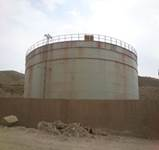Figure 2. A view of the 10 thousand cubic meter tank of Mashhad Cement Company.

The thickness of the wall, ceiling and floor of the steel tank in 5, 10 and 15 centimeter forms were studied. Also the weight of TNT caused by the blast was considered 10 tons and the distances of the blast occurrence were assumed 15, 25, 50, 100 and 200 meters.

Considering that the blast intended occurs on the surface, the waves at the blast surface because of the reflection from the ground are retrofitted and as in this mode the explosives are so close to or in contact with the ground surface, therefore, the blast waves are released and spread in a semi-spherical form.

(Z) the scaled distance, is defined as follows:

Z = R/(W)1/3               (1)

In this equation (W) is TNT equivalent weight in terms of kilo grams for the explosives intended and (R) is the distance of the materials to the target (Stand-off) in terms of meter. This parameter was first stated independently by B. Hopkinson in 1915 and then by C. Cranz in 1926 .

The primary pressure is generated at a distance from the blast centroid is much more than the atmospheric pressure. This pressure generated is called "Over pressure", which is shown with (Ps) and (P so) is the maximum value. The period of time, which (P so) is added to the pressure of the environment is very sudden, as it can be considered as instantaneous.

As it is seen in figure 5, the pressure wave expands radially from the blast centroid with a reducing speed, which this speed is always more than the speed of the sound in the environment. The pressure changes generated in every point, which is at a desired distance from the blast centroid is as shown in figure 5.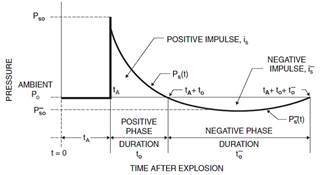Figure 3. The pressure graph of blast wave of the time [].

In a moment such as "Arrival Time" (tA), which is the time of the wave ascending, it will reach the point intended and the amount of pressure will increase instantaneously to the amount of (P so) and then it will decrease to reach the environment pressure. This decreasing process is done in duration of (to). This duration from (tA) to (to) is called "Positive phase". After that the negative phase will start and the pressure will reach below atmosphere. The duration of negative phase is shown with (to-), which is usually more than positive phase. however, due to the slight negative phase; it can be disregarded in designing and analyzing.

To mitigate the over pressure with the time the following exponential equation is suggested:

P(s) / Pso = [1- (t-tA/to) ]e –(t-tA)/to        (2)

To calculate (P so) an equation by Kinney & Graham (1985) has been suggested, which is as the following form of equation :

Pso/Pa={808[1+(z/4.5)2]}/{√1+(z/0.048)2.√1+(z/1.32)2.√1+(z/0.32)2}   (3)

(P so) is the peak over pressure and (Pa) is equivalent to the atmospheric pressure in terms of (kg/cm2), which is usually considered equivalent to 100 KN per cubic meter. The cases which have been stated so far are in relation to the pressure increase in one point in the open air, however, if at this point there is a level or a rigid structure, the over pressure maximum amount will not be (P so) anymore , when the pressure primary wave hits a rigid surface, it is reflected from the surface, therefore, the waves are compressed on the surface momentarily, so the peak pressure generated on the surface is definitely more than the amount of the pressure, which is generated in the absence of a liquid existing at that point. The problem can be expressed in this way that when a structure is located in the direction of a blast wave, the interaction between the air moving and the body of the structure results in reducing the waves velocity and the kinetic energy of the particles in the air suddenly reaches zero, thus an increasing loading will be exerted to the structure. The striking pressure has been presented with (P r), which its amount is subject to the amount of (P so) at the same point .

The amount of (P r) is obtained from the following equation:

Pr = 2Pso (4Pso+7Pa)/(Pso+7Pa)        (4)

This equation was obtained by Rankine (1870), assuming that the air behaves like an ideal gas. In this equation (P r) in comparison to the pressure generated on the surface of the structure is more at a point, where the connecting line of that point to the centroid of the blast is perpendicular to the surface of the structure.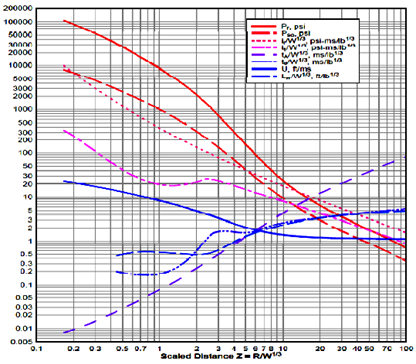Figure 4. Shock wave parameters of the positive phase for spherical surface blasts .

The duration of the positive phase (to) is a function of (P so) and also the amount of the total energy released in the blast. The equation to determine (to) was presented by Kinney & Graham (1985) as follows:

t/3√w={980[1+(z/0.45)10]}/{[1+(z/0.02)3].[1+(z/0.75)6].[1+(z/6.9)2]-1}     (5)

Where (W) is the weight of the explosive and (Z) is equal to the distance scaled, while the positive phase duration is obtained in terms of Millisecond.

As well as the theoretical equations, which have been stated so far to calculate the blast load parameters, some graphs have also been provided based on the practical tests in this regard. In figure 4, the graph of different parameters have been shown in the form of a function to the distance scaled of (Z) for the positive phase. These curves are related to the surface blast .

Figure 5, The loading history considered is seen in a mode, which the distance of the blast from the structure is 100 meters.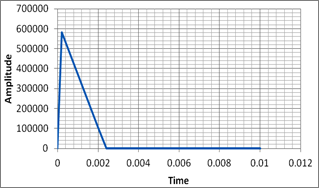Figure 5. The history of explosive load.

To study the behavior of the structure under explosive loading, a 3D form and Abaqus V.6 software are used. In this research, considering the foundation of this structure, which is from concrete, the pressure resistance intended for it is assumed 35 Mega Pascal, the Poisson ratio 0.2 and elasticity modulus 20 and 59 Giga Pascal and the relative density 2400 kg per cubic meter. The concrete property in the plastic area has been presented in tables 1 and 2.

Table 1. The values of stress, strain and degradation of plastic concrete in tensile mode.

 Parameter degradation in stretch Strain cracking Tensile strength (Mpa) 0.00 0.000000 5.3 0.25 0.000176 5.81 0.99 0.001539 0.58

Table 2. The values of stress, strain and degradation of plastic concrete in pressure.

 Parameter degradation in pressure Non- elastic strain Compressive strength(Mpa) 0.000 0.00000 17.5 0.112 0.00038 25.7 0.429 0.00189 34.9 0.466 0.00218 35 0.701 0.00456 28

To make a model of the tank, which is made of steel, in the area of the elasticity, the elastic modulus is assumed 210 Giga Pascal, Poisson coefficient 0.3 and relative density 7800 kg per cubic meter and the properties of steel in the plastic area for blast analysis can also be seen in table 3.

Table 3. The values of steel actual stress and strain.

 Plastic strain Real tension (Mpa) 0.000 300 0.025 350 0.100 375 0.200 394 0.350 400

In figure 6, Mazut 10 thousand cubic meter tank model made can be seen, the ceiling, floor and wall sections, which are of the same type, have been considered to be integrated.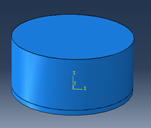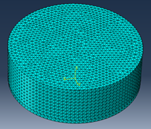Figure 6. Tank model made in Abaqus software.

Figure 7, The graph related to displacement shows the ratio of the time for various distances of blast to the structure.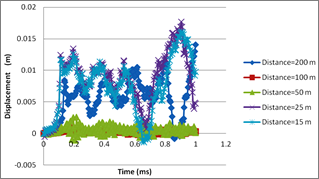a)Thickness of 5 centimeters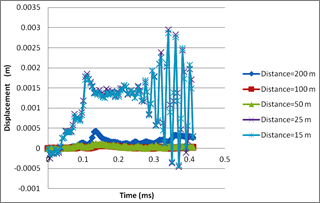b)Thickness of 10 centimeters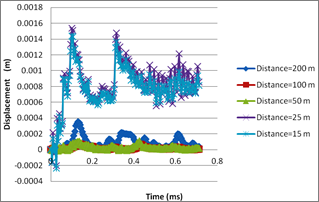c)Thickness of 15 centimeters

Figure 7. Graph of displacement to the time for various distances of the blast site from the structure.

Figure 7 shows that with the increase of the blast distance to the structure, the effect of the blast wave will be mitigated. Also in low thicknesses, the displacements are more than the mode, which the thickness of the tank steel wall is more.

In figure 8, a view of the structure deformation in the vertical direction, while the thickness of the tank is 5 centimeters and the distance of the tank is 25 meters from the from the blast site, has been shown.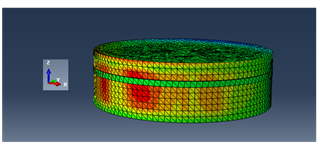a)A general overview of the model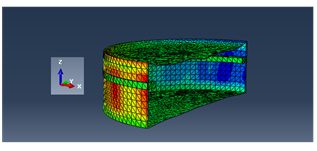b)A ross-sectional overview of the model

Figure 8. A deformation overview of the model under explosive load.

The displacement graph in terms of time for the thicknesses of 5, 10 and 15 centimeters of the tank in a mode, which the distance of the explosive site from the structure is 15 and 100 meters, which has been presented in figure 9, it can be realized that the more the thickness of the tank wall becomes, the less the blast effect will be and the thickness also plays an important role in the displacements.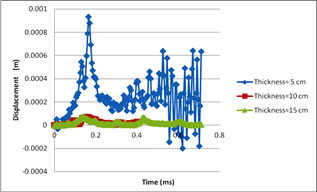a)Distance of the explosion from the structure, 100 meters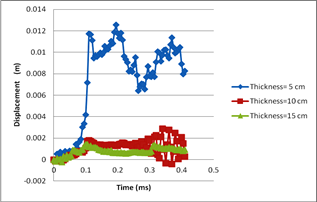b)Distance of the explosion from the structure, 15 meters

Figure 9. Displacement in terms of time for 15 and 100 meters distances of the explosion.

Energy graph for the whole structure can also be seen in figure 10.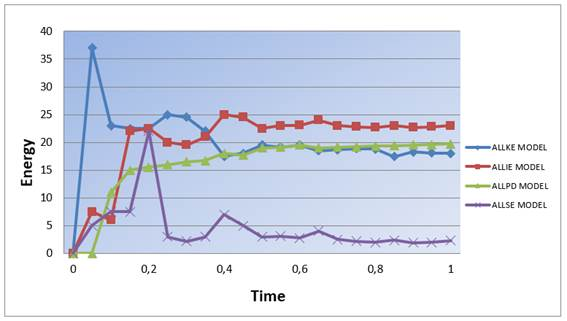Figure 10. The graph of energy of the structure.

6. Discussion and Conclusion

The 10 thousand cubic meter tank centroid made is with the diameter of 34 centimeters and the height of 10 meters, while its foundation is ring shape with an interior dimension of 30 meters, which can be seen with the help of Abaqus 3D software. The structure was analyzed in the mode of explosive loading. The type of explosive has been assumed TNT and its weight has been assumed 10 tons. The blast site distances intended from the structure, 15, 25, 50, 100 and 200 meters for the thicknesses of 5, 10 and 15 centimeters of the tank were exerted in the calculations. The conclusions indicated that with the increase of the blast distance from the tank, the intensity of the wave obtained from the blast is strongly reduced, This is while the thickness considered for the tank plays a significant role in the sustainability and mitigation of the displacements and with the increase of the thickness of the steel tank, the safety coefficient of the structure against blast loads will increase.

References

1. The most comprehensive reference book used Abaqus. Sohail Srvshnya and Nima Paradise..pp,124-150.
2. E. Mohtashami, Sinai, Sinai, Ahmed Shoshtari. "The assessment of the behavior of steel frames against explosion charges" Fifth National Congress of Civil Engineering, University of .p,45,58,67.
3. Mahmoud Imam, Ahmad Shoshtari. "Blast effects on reinforced concrete buildings earthquake resistant," the Sixth National Congress of Civil Engineering. Semnan University in .p34,86,111.
4. National Building Regulations, "Section 21: Civil Defense".pp3-6.
5. Bing, L.,Tso Chien,P. and Anan.,N.,"A case study of the effect of cladding panels on the response of reinforced concrete frames subjected to distant blast loading". Elsevier,Singapore.pp,130-142.
6. Hesamoddin forearm Yousefi, Shariatmadari. "Check flexible filler walls and reinforced concrete structures under load in response to lateral stiffness remote detonation" thesis, Ferdowsi University..p,75,120.
7. Kinney, G.F. and Graham, K.J. , Explosive Shocks in Air, Springer-Verlag, New York, 2nd edn.
8. M.Y.H.Bangash"Shock, Impact and Explosion"Structural Analysis and Design. page 430.
9. Donald O. Dusenberry "Handbook for Blast Resistant Design of Buildings" page176.
10. A.Remennikov."The state of the art of explosive loadscharacterisation" .p12.
11. Emily L. Guzas. and Christopher J. Earls "Air blast load generation for simulating structural response".pp433-437.

 Contents 1. 2. 3. 4. 5. 6.
Article ToolsAbstractPDF(2418K)